Courses

# Diffusivity In Solids And Its Applications Chemical Engineering Notes | EduRev

## Chemical Engineering : Diffusivity In Solids And Its Applications Chemical Engineering Notes | EduRev

The document Diffusivity In Solids And Its Applications Chemical Engineering Notes | EduRev is a part of the Chemical Engineering Course Mass Transfer.
All you need of Chemical Engineering at this link: Chemical Engineering

2.7 Diffusivity in Solids and its Applications
In many processes such as drying, adsorption and membrane separations require the contact of gases or liquids with solids. Diffusion occurs in these cases in the solid phase and the diffusion mechanism is not as simple as in the case of gases or liquids. However, it is possible to describe by the Fick‟s law used in the case of fluids. If the diffusivity is independent of concentration and there is no bulk flow, the steady state molar flux (NA) in the Z direction is given by Fick‟s law as follows: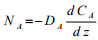(2.76)
where DA is the diffusivity of A through the solid. Integration of the above Equation gives diffusion through a flat slab of thickness z: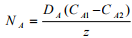(2.77)
where CA1 and CA2 are the concentrations at two opposite sides of the slab. This is similar expression for diffusion obtained for fluids under identical situation. For other solid shapes the general Equation for rate of diffusion (w) is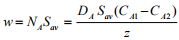(2.78)

where Sav is the average cross section for diffusion.

2.7.1 Mechanism of diffusion in solids and its application
The diffusion of solutes through the solids plays an important role in many processes such as heterogeneous catalytic reactions. The structure of solid and interaction with the solutes are important for the rate of diffusion.
(a) Diffusion in porous solids
The solid sometimes may act as porous barrier or as porous catalyst pellets and is normally surrounded by a single body of fluid. The inward or outward movement of the solutes through the pores of the solid is mainly by diffusion. This movement may occur inside the pore or at the surface of the adsorbed solute.

(b) Diffusion inside pore
At low pressure the mean free path of the molecules may be larger than the diameter of the passage when the diffusion occurs inside the fine pores of the solid. The collision with wall becomes important compared to collision among molecules. The diffusion of this kind is known as „Knudsen diffusion‟. To quantify Kundsen diffusivity a simple Equation based on kinetic theory of gases was proposed as follows: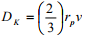(2.79)
where rp radius of passage and v is the average velocity of the molecules due to their thermal energy which is defined as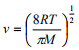(2.80)
Here T is the temperature in K and M is the molecular weight. The flux due to Knudsen diffusion is similar to Fick‟s law: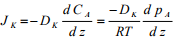(2.81)

(c) Surface diffusion
The diffusion of adsorbed molecules on the surface due to concentration gradient is kwon as surface diffusion. If the fractional coverage of the surface is less than unity then the some of the active sites remain empty. Adsorbed molecule having energy greater than the energy barrier tends to migrate to an adjacent vacant site. This migration is visualized to occur by „hoping‟ mechanism. The flux due to surface diffusion may be written similar to Fick‟s law: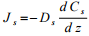(2.82)
where Ds is the surface diffusion coefficient (m2/s) and Cs is the surface concentration of the adsorbed molecules (kmol/m2 ). Js is the number of moles transported across unit distance on the surface normal to the direction of transport (kmol/m.s).

(d) Diffusion through polymers
The diffusion of solutes through polymeric solids is more like diffusion in liquids, particularly for the permanent gases. The gas dissolves into the solid exposed to the gas and usually described by Henry‟s law. The gas then diffuses from high to low pressure side. Hence, high pressure is applied to increase gas concentrations, thus increasing respective solubility (difference in solubility is the key feature) of gases. The polymeric chains are in a state of constant thermal motion and diffusing molecule move from one location to the adjacent location due to the potential barrier. An Arrhenius type Equation may be applied for the temperature dependency of the diffusion coefficient in polymers.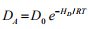(2.83)
where HD is the energy of activation and D0 is a constant. For the permanent gases the typical diffusivity value is in the order of 10-10 m2/s.

Example Problem 2.1:
A test tube, 1.5 cm in diameter and 18 cm long, has 0.4 gm camphor (C10H16O) in it. How long will it take for camphor to disappear? The pressure is atmospheric and temperature is 200C. The sublimation pressure of camphor at this temperature is 97.5 mm Hg; diffusivity of camphor can be estimated by using Fuller‟s Equation: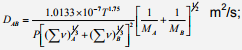where, T in K; P in bar, MA, MB are molecular weights of A and B, respectively.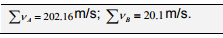Solution2.1:
MA=152.128,
MB=28.9,
T=293K,
P=1.013 bar (1atm)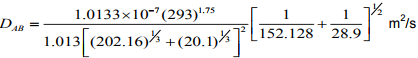DAB=5.644�10-6 m2/s
pAo = 97.5 mmHg =0.1299 bar
pA1 = 0 bar
/=12 cm=0.12 m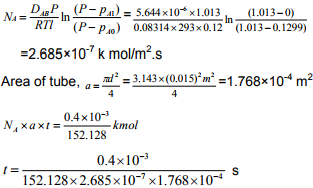=5.539�104s
t =15.39 hr

Example Problem 2.2:
A mixture of He and N2 gas is contained in a pipe at 250C and 1 atm. total pressure which is constant throughout. At one end of the pipe, partial pressure of He is 0.6 atm. and at the other end (0.2 m) it is 0.2 atm. Calculate the steady state flux of He if DAB of He-N2 mixture can be estimated by using Fuller‟s Equation with
∑v=2.88m/s; and ∑vB =17.9m/s.

Solution 2.2: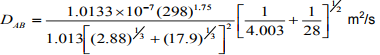DAB=7.035�10-5 m2/s
pA0 = 0.6 atm
pA1 = 0.2 atm
/=0.2 m
T=298K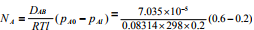=5.68�10-6 k mol/m2.s

Example Problem 2.3: MeOH (A) is separated from aqueous solution by distillation. At a section of column, vapor phase contains 0.76 mole fraction MeOH and liquid phase has 0.6 mole fraction. Temperature of the section is 71.20C and total pressure is 1 atm. throughout 1 mm thick vapor film. If molar latent heat of vaporization of MeOH is 8787 K Cal/K mol and that of water (B) is 10039 K Cal/K mol at the given temperature. Calculate MeOH and water vapor flux.
Given: If mole fraction of MeOH in liquid is 0.6, equilibrium vapor will be 0.825. Vapor phase diffusivity of MeOH, DAB=1.816�10-5 m2/s.

Solution 2.3: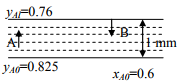NA∆HA = - NB∆HB
NA�8787 = -NB�10039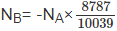NB=-0.8753NA
Given data,
DAB=1.816 �10-5 m2/s,
P=1 atm,
R=0.0823 m3atm/kmol
T=344.2 K,
/=1�10-3 m,
yA0=0.825,
yA1=0.76
We have,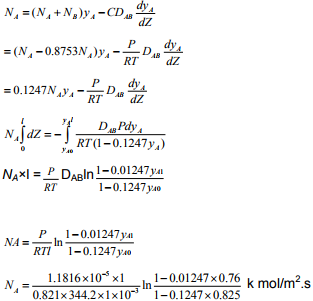=4.65�10-5 k mol/m2.s
NB=-0.8753�4.65�10-5 k mol/m2.s
=-4.07�10-5 k mol/m2.s

Offer running on EduRev: Apply code STAYHOME200 to get INR 200 off on our premium plan EduRev Infinity!

,

,

,

,

,

,

,

,

,

,

,

,

,

,

,

,

,

,

,

,

,

;